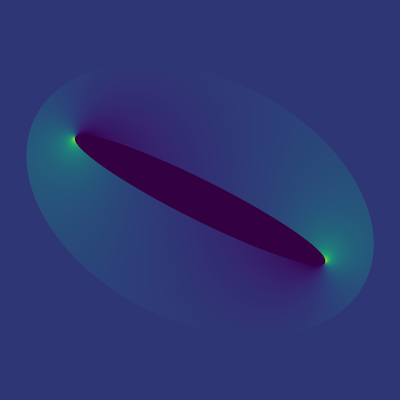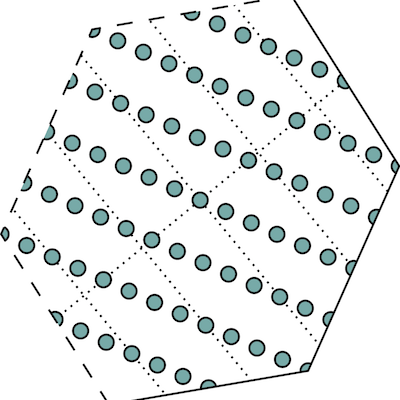# Homogenization in Translation Invariant Periodic Spaces

This project aims to investigate the discretization of elliptic partial differential equations with periodic boundary conditions on anisotropic spaces of translates. Of special interest are the influence of the anisotropic sampling lattice, the choice of the translation invariant space, convergence results and the investigation of periodic wavelets.

## References

1. BergmannMerkert-2020Bergmann, R., Merkert, D.2020FFT-based homogenization on periodic anisotropic translation invariant spaces
Applied and Computational Harmonic Analysis481266–292

In this paper we derive a discretisation of the equation of quasi-static elasticity in homogenization in form of a variational formulation and the so-called Lippmann-Schwinger equation, in anisotropic spaces of translates of periodic functions. We unify and extend the truncated Fourier series approach, the constant finite element ansatz and the anisotropic lattice derivation. The resulting formulation of the Lippmann-Schwinger equation in anisotropic translation invariant spaces unifies and analyses for the first time both the Fourier methods and finite element approaches in a common mathematical framework. We further define and characterize the resulting periodised Green operator. This operator coincides in case of a Dirichlet kernel corresponding to a diagonal matrix with the operator derived for the Galerkin projection stemming from the truncated Fourier series approach and to the anisotropic lattice derivation for all other Dirichlet kernels. Additionally, we proof the boundedness of the periodised Green operator. The operator further constitutes a projection if and only if the space of translates is generated by a Dirichlet kernel. Numerical examples for both the de la Vallée Poussin means and Box splines illustrate the flexibility of this framework.

@article{BergmannMerkert-2020, number = {1}, pages = {266–292}, doi = {10.1016/j.acha.2018.05.003}, author = {Bergmann, R. and Merkert, D.}, eprint = {1701.04685}, year = {2020}, eprinttype = {arXiv}, volume = {48}, journaltitle = {Applied and Computational Harmonic Analysis}, title = {{FFT}-based homogenization on periodic anisotropic translation invariant spaces}, abstract = { In this paper we derive a discretisation of the equation of quasi-static elasticity in homogenization in form of a variational formulation and the so-called Lippmann-Schwinger equation, in anisotropic spaces of translates of periodic functions. We unify and extend the truncated Fourier series approach, the constant finite element ansatz and the anisotropic lattice derivation. The resulting formulation of the Lippmann-Schwinger equation in anisotropic translation invariant spaces unifies and analyses for the first time both the Fourier methods and finite element approaches in a common mathematical framework. We further define and characterize the resulting periodised Green operator. This operator coincides in case of a Dirichlet kernel corresponding to a diagonal matrix with the operator derived for the Galerkin projection stemming from the truncated Fourier series approach and to the anisotropic lattice derivation for all other Dirichlet kernels. Additionally, we proof the boundedness of the periodised Green operator. The operator further constitutes a projection if and only if the space of translates is generated by a Dirichlet kernel. Numerical examples for both the de la Vallée Poussin means and Box splines illustrate the flexibility of this framework. }, }
2. BergmannMerkert-2018Bergmann, R., Merkert, D.2018A framework for FFT-based homogenization on anisotropic lattices
Computers & Mathematics with Applications.761125–140

In order to take structural anisotropies of a given composite and different shapes of its unit cell into account, we generalize the Basic Scheme in homogenization by Moulinec and Suquet to arbitrary sampling lattices and tilings of the $d$-dimensional Euclidean space. We employ a Fourier transform for these lattices by introducing the corresponding set of sample points, the so called pattern, and its frequency set, the generating set, both representing the anisotropy of both the shape of the unit cell and the chosen preferences in certain sampling directions. In several cases, this Fourier transform is of lower dimension than the space itself. For the so called rank-$1$-lattices it even reduces to a one-dimensional Fourier transform having the same leading coefficient as the fastest Fourier transform implementation available. We illustrate the generalized Basic Scheme on an anisotropic laminate and a generalized ellipsoidal Hashin structure. For both we give an analytical solution to the elasticity problem, in two- and three dimensions, respectively. We then illustrate the possibilities of choosing a pattern. Compared to classical grids this introduces both a reduction of computation time and a reduced error of the numerical method. It also allows for anisotropic subsampling, i.e. choosing a sub lattice of a pixel or voxel grid based on anisotropy information of the material at hand.

@article{BergmannMerkert-2018, issue = {1}, pages = {125–140}, doi = {10.1016/j.camwa.2018.04.008}, author = {Bergmann, R. and Merkert, D.}, eprint = {1605.05712}, year = {2018}, eprinttype = {arXiv}, volume = {76}, journaltitle = {Computers & Mathematics with Applications.}, title = {A framework for {FFT}-based homogenization on anisotropic lattices}, abstract = { In order to take structural anisotropies of a given composite and different shapes of its unit cell into account, we generalize the Basic Scheme in homogenization by Moulinec and Suquet to arbitrary sampling lattices and tilings of the $d$-dimensional Euclidean space. We employ a Fourier transform for these lattices by introducing the corresponding set of sample points, the so called pattern, and its frequency set, the generating set, both representing the anisotropy of both the shape of the unit cell and the chosen preferences in certain sampling directions. In several cases, this Fourier transform is of lower dimension than the space itself. For the so called rank-$1$-lattices it even reduces to a one-dimensional Fourier transform having the same leading coefficient as the fastest Fourier transform implementation available. We illustrate the generalized Basic Scheme on an anisotropic laminate and a generalized ellipsoidal Hashin structure. For both we give an analytical solution to the elasticity problem, in two- and three dimensions, respectively. We then illustrate the possibilities of choosing a pattern. Compared to classical grids this introduces both a reduction of computation time and a reduced error of the numerical method. It also allows for anisotropic subsampling, i.e. choosing a sub lattice of a pixel or voxel grid based on anisotropy information of the material at hand. }, }
3. BergmannMerkert-2017Bergmann, R., Merkert, D.2017Approximation of periodic PDE solutions with anisotropic translation invariant spaces
in: 2017 International Conference on Sampling Theory and Applications (SampTA)396-399

We approximate the quasi-static equation of linear elasticity in translation invariant spaces on the torus. This unifies different FFT-based discretisation methods into a common framework and extends them to anisotropic lattices. We analyse the connection between the discrete solution spaces and demonstrate the numerical benefits. Finite element methods arise as a special case of periodised Box spline translates.

@inproceedings{BergmannMerkert-2017, booktitle = {2017 International Conference on Sampling Theory and Applications (SampTA)}, author = {Bergmann, R. and Merkert, D.}, doi = {10.1109/SAMPTA.2017.8024347}, pages = {396-399}, title = {Approximation of periodic PDE solutions with anisotropic translation invariant spaces}, abstract = { We approximate the quasi-static equation of linear elasticity in translation invariant spaces on the torus. This unifies different FFT-based discretisation methods into a common framework and extends them to anisotropic lattices. We analyse the connection between the discrete solution spaces and demonstrate the numerical benefits. Finite element methods arise as a special case of periodised Box spline translates. }, year = {2017}, }